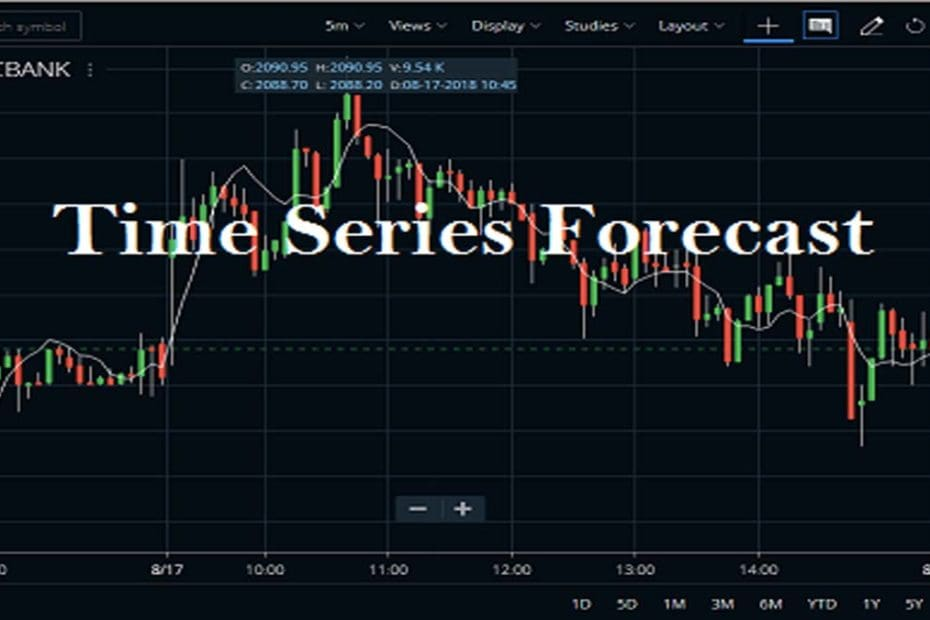Home » Blog » Trading » Technical Indicators » Time Series Forecast Indicator Settings, Trading Strategy

# Time Series Forecast Indicator Settings, Trading Strategy

• Technical IndicatorsThe Time Series Forecast indicator (TSF) shows the statistical trend of a security’s price over a specified time period. This indicator is referred to as a moving linear regression that is similar to a moving average. The Time Series Forecast (TSF) indicator is based upon a regression-based forecast model. It predicts future price action based on past events.

## Top FAQs about Time Series Forecast Indicator

What is Time Series Forecast Indicator?

This indicator basically reflects the statistical trends over a specific period of time. And it can give an indication of the current trend continuation. The indicator fits itself to the underlying price data instead of average prices. Therefore, tends to be more responsive to sudden changes in price compared to a moving average.

How to use Time Series Forecast Indicator?

The usage of this indicator is straight. When the Price moves above the TSF line this determines a bullish trend. On the other side, when the Price falls below the TSF line, this indicates a bearish trend. This is the basic strategy. Apart from this, there are many useful ones that are described later in this article.

How to Calculate Time Series Forecast Indicator?

This indicator is a linear regression calculation. It plots each bar’s current regression value by using the least square fit method.

## How To Attach Time Series Forecast Indicator for Technical Analysis?

Traders can know more about this TSF indicator, then they can find it in the STUDIES section of Zerodha Kite. It is also available in Kite mobile App. The default Period is 14. The default Field is close. You can set the value of it to open, high, low, close or hl/2, hlc/3, hlcc/4, and ohlc/4. Traders can use this indicator on any time frame charts likes daily, weekly, monthly, or intraday. You can also check the image below to understand how to attach the TSF indicator to the HDFC Bank share price chart.

Here, is the next example, Upstox Pro. Here, just open a chart and search for the indicator. After that, click on apply. After clicking on the apply, the TSF comes over the price chart.

## Key Points on Time Series Forecast Indicator

• Traders can interpret this indicator in the same way as other moving averages.
• This indicator is useful for smoothing noise out of the price movements with the analysis of moving averages traders can gain a general idea of where the underlying trend is headed.
• TSF formula creates a visual display of the statistical trend of the stock price over a specified time period.
• Where the simple moving average is the trend of historical closing prices for a time period.
• The TSF is the trend of stock prices based on the linear regression of closing prices.
• The TSF does not create a straight linear regression trend line. Rather the TSF plots the last point of multiple linear regression trend lines.

## Conclusion

An indicator is an interesting tool for traders who are tired of trading lag between the underlying prices of a stock and the moving average. This is differentiated moving averages by not only be more responsive to price changes but also forecasting future price changes. This is depending on the traders’ trading strategy in using the least-squares regression technique. Compare to all trading indicators, we always suggest that the trader experiment with different time frames to find the one that suits traders’ trading and risk profile. Also, traders can use multiple indicators to provide confirmation for buy and sell signals.